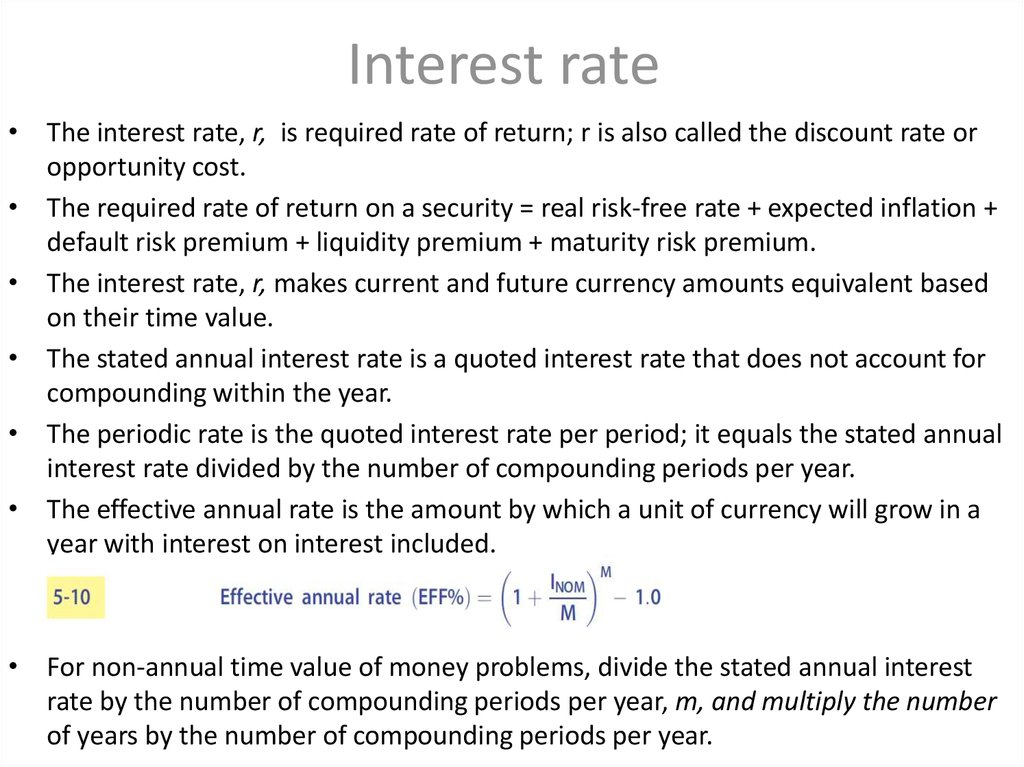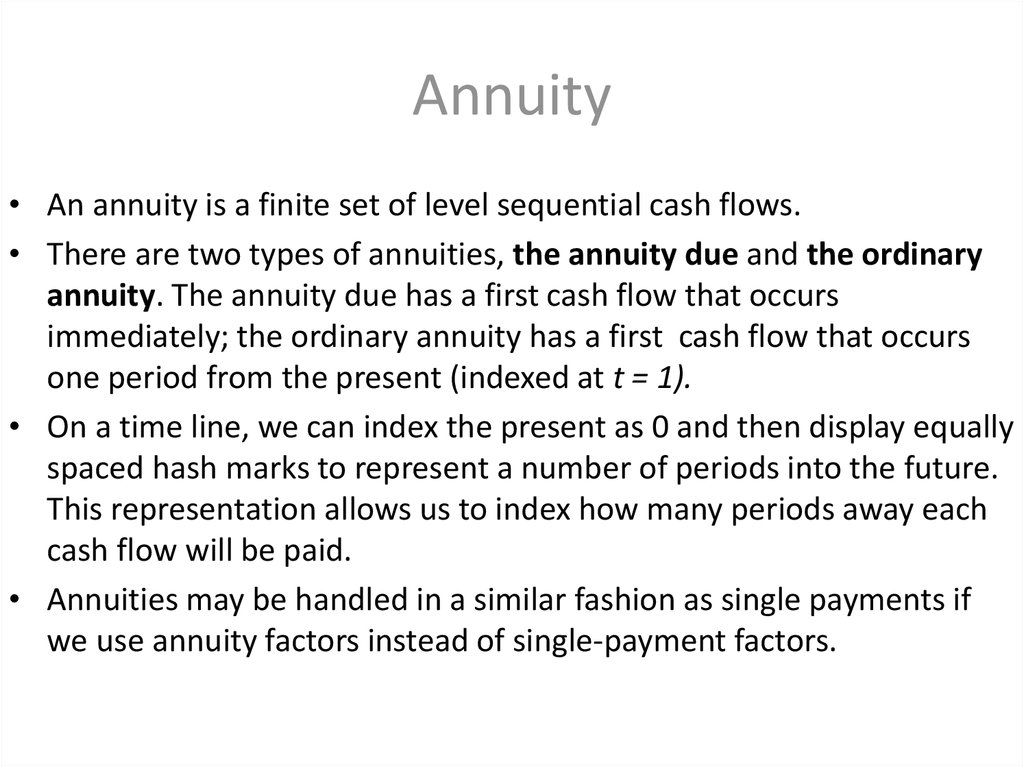# Time value of money

## 1. 1.1Time value of money

By Dias Kulzhanov

## 2. Interest rate

• The interest rate, r, is required rate of return; r is also called the discount rate or
opportunity cost.
• The required rate of return on a security = real risk-free rate + expected inflation +
• The interest rate, r, makes current and future currency amounts equivalent based
on their time value.
• The stated annual interest rate is a quoted interest rate that does not account for
compounding within the year.
• The periodic rate is the quoted interest rate per period; it equals the stated annual
interest rate divided by the number of compounding periods per year.
• The effective annual rate is the amount by which a unit of currency will grow in a
year with interest on interest included.
• For non-annual time value of money problems, divide the stated annual interest
rate by the number of compounding periods per year, m, and multiply the number
of years by the number of compounding periods per year.

## 3. Annuity

• An annuity is a finite set of level sequential cash flows.
• There are two types of annuities, the annuity due and the ordinary
annuity. The annuity due has a first cash flow that occurs
immediately; the ordinary annuity has a first cash flow that occurs
one period from the present (indexed at t = 1).
• On a time line, we can index the present as 0 and then display equally
spaced hash marks to represent a number of periods into the future.
This representation allows us to index how many periods away each
cash flow will be paid.
• Annuities may be handled in a similar fashion as single payments if
we use annuity factors instead of single-payment factors.

## 5. Future Value/Present Value of an Ordinary Annuity

*FVAn- future value for an ordinary annuity
*PVAn- present value for an ordinary
annuity

## 6. Perpetual annuities(PV annuities with infinite lives)

PV and FV of Uneven Cash Flow series
It is not uncommon to have applications in investments and corporate finance
where it is necessary to evaluate a cash flow stream that is not equal from
period to period.